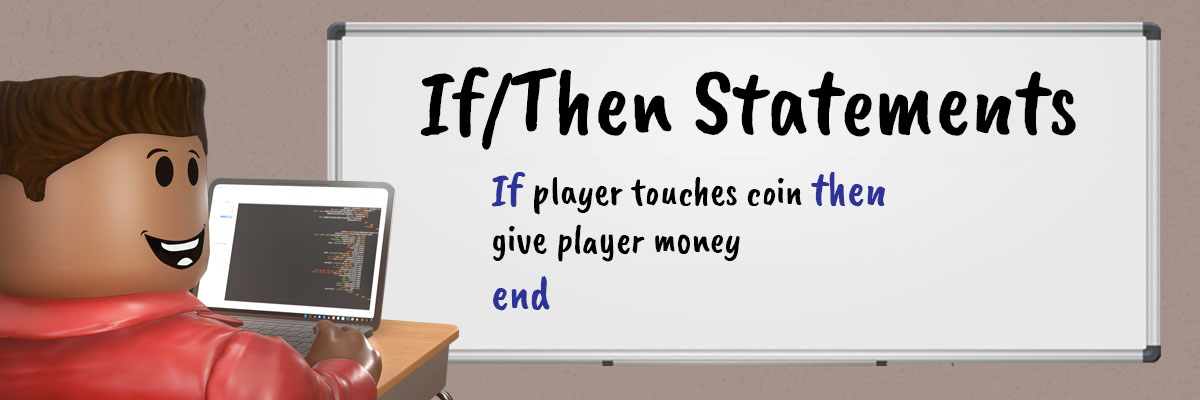# Using if/then StatementsAs you make games, you will find yourself wanting to make cause-effect relationships like the following:

• If a player scores 10 points, then they win the game.
• If a player has a power-up, then they can run super fast.
• If a player says “Happy Birthday” in chat, then sparkles appear.

All of these relationships need a conditional statement, lines of code that only run if certain conditions are true. One type of conditional statement is an if/then statement that checks to see if something is true before running instructions.

## Practicing Conditional Statements

In this code example, a part will change transparency whenever the statement is true. Let’s practice with an obviously true statement like `3+3 = 6`. Since this statement is true, the next line of code will run.

### Set up the Part and Script

1. In ServerScriptService, create a new script named IfThenScript.

2. Create a new part named IfThenPart.

3. Delete `Hello World` in the script and write a comment at the top.### Code the Condition

The condition in this case is if 3 + 3 is equal to 6, then something else will happen. To create this condition:

1. Type lowercase `if 3+3`2. After `3+3`, type `==`##### Difference between == and =
• == compares values. It’s used for if statements.
• = sets values for variables

One way to remember which is which is that “compare” has two syllables, and two symbols. “Set” is one syllable and one symbol.

1. After `==`, type `6`. This is what you’ll check against. If you’re using other numbers, make sure the condition is true, like `10 - 4 == 6`.

### Code the Statement

After making the condition, the script needs a statement that’ll happen when that condition is true. In this case, the part will change transparency whenever the condition is true.

1. After if `3+3 == 6`, type `then`2. Press Enter to autocomplete the if statement with `end`3. Between `then` and `end`, type `game.Workspace.BrickName.Transparency = .5`1. Test your code. Check that the part has changed transparency.• Make sure `if`, `then`, and `end` are lowercase.
• Use `==`, not a single `=`.
• Make sure that the code where you change the part’s transparency is between `if` and `end`. If not, the statement may still work, but technically won’t be a part of the if/then statement.

## Check a False Condition

Now, purposely change the statement to see what happens when the math equation is false.

1. Change `if 3+3 == 6 then` to `if 3+3 == 10 then`

2. Test your code now. The part will no longer change transparency because the statement is no longer true.## Making Other Comparisons

Instead of checking if two numbers are equal, you can also multiply, add, subtract, or make other comparisons. Depending on the games you make, one might be more useful than another.

Instead of using `==`, complete these challenges using the Math and Comparison Operators table below:

• Checks if a number is less than 10
• Checks if a number is greater than or equal to 200

Checks if a number is less than 10:
`if myNumber < 10`

Checks if a number is is greater than or equal to 200:
`if myNumber >= 200`

Symbol Meaning
- Subtraction
* Multiplication
/ Division

### Comparison Operators

Symbol Meaning
`==` Is equal to
`~=` Is not equal to
`<=` Is less than or equal to
`>=` Is greater than or equal to
` < ` Is less than
` > ` Is greater than

These documents are licensed by Roblox Corporation under a Creative Commons Attribution-NonCommercial-ShareAlike 4.0 International License. Roblox, Powering Imagination, and Robux are trademarks of Roblox Corporation, registered in the United States and other countries.

Previous Multiple Parameters and Arguments Next Traps with if/then Statements